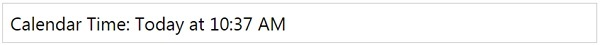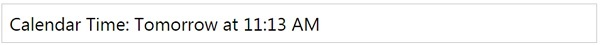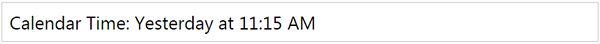# MomentJS - Calendar Time

This method displays the time difference to given reference time and the Calendar time.

## Syntax

```moment().calendar();
moment().calendar(referenceTime);
moment().calendar(referenceTime, formats);
```

## Example 1

```var changeddate = moment().calendar();
```

## Output## Example 2

We have discussed chaining method in earlier chapter, so we can use the add/subtract methods with moment to get the calendar value as shown below −

```var changeddate = moment().add(24, 'h').calendar();
```

## Output## Example 3

```var changeddate = moment().subtract(24, 'h').calendar();
```

## Outputmomentjs_formatting_date_and_time.htm